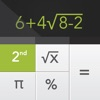# Graphing Calculator + | 5.in.1 Advanced Math Equation Solve.r for Graph.ic, Linear Algebra, Trig.onometry, Calc.ulus & more! - By xNeat.com - Lumos Educational App Store31
Price -\$4.99
\$4.99

#### DESCRIPTION:

Graphing Calculator+ is an iPhone/iPad calculator designed with simplicity, usability, and beauty in mind... After installing Graphing Calculator+ you get access to 5 different calculators: 1- Graphing calculator 2- Handwriting calculator 3- Scientific calculator 4- Quadratic & Cubic equation solver 5- System of linear equations solver FEATURES: - Elegant & intuitive interface - Supports handwriting - Displays both the equation & the result at the same time - Supports many scientific functions - Solving system of linear equations 2x Ã¢â¬â y = 9 3x + 4y = Ã¢â¬â14 - Solving

#### OVERVIEW:

Graphing Calculator + | 5.in.1 Advanced Math Equation Solve.r for Graph.ic, Linear Algebra, Trig.onometry, Calc.ulus & more! - By xNeat.com is a free educational mobile app By xNeat.com.It helps students in grades 5 practice the following standards 5.G.A.1,5.G.A.2,.

This page not only allows students and teachers download Graphing Calculator + | 5.in.1 Advanced Math Equation Solve.r for Graph.ic, Linear Algebra, Trig.onometry, Calc.ulus & more! - By xNeat.com but also find engaging Sample Questions, Videos, Pins, Worksheets, Books related to the following topics.

1. 5.G.A.1 : Use a pair of perpendicular number lines, called axes, to define a coordinate system, with the intersection of the lines (the origin) arranged to coincide with the 0 on each line and a given point in the plane located by using an ordered pair of numbers, called its coordinates. Understand that the first number indicates how far to travel from the origin in the direction of one axis, and the second number indicates how far to travel in the direction of the second axis, with the convention that the names of the two axes and the coordinates correspond (e.g., x-axis and x-coordinate, y-axis and y-coordinate)..

2. 5.G.A.2 : Represent real world and mathematical problems by graphing points in the first quadrant of the coordinate plane, and interpret coordinate values of points in the context of the situation. .

5

#### STANDARDS:

5.G.A.1
5.G.A.2

Developer: xNeat.com

Software Version: 2.1.3

Category: Education

### RELATED APPSEdSearch WebSearch